Anúncio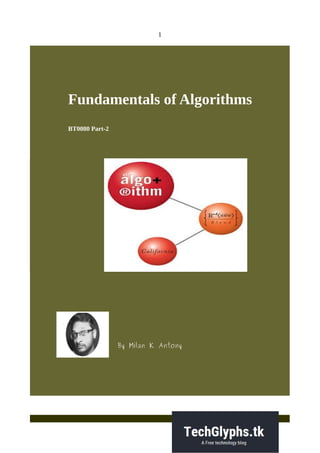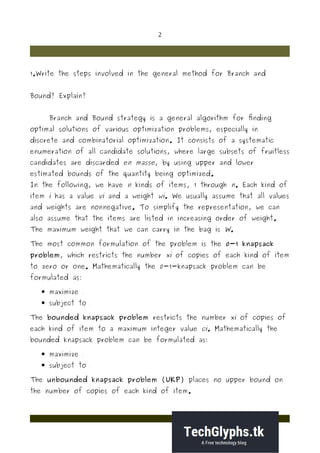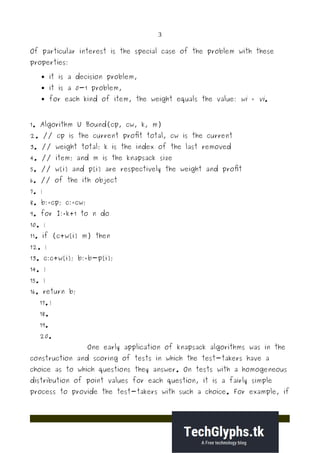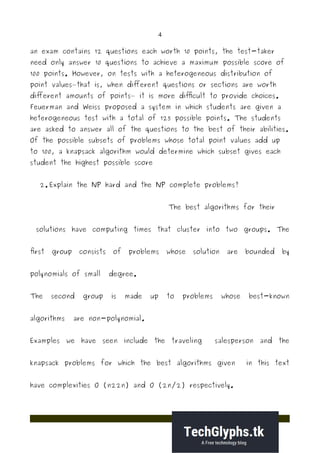Anúncio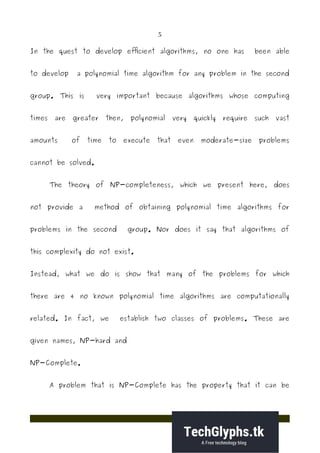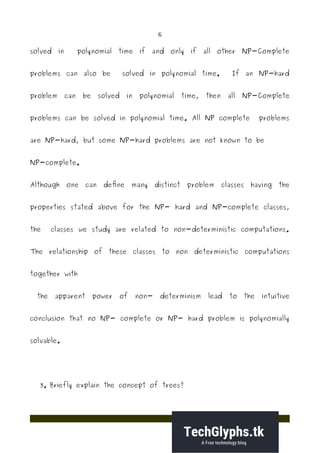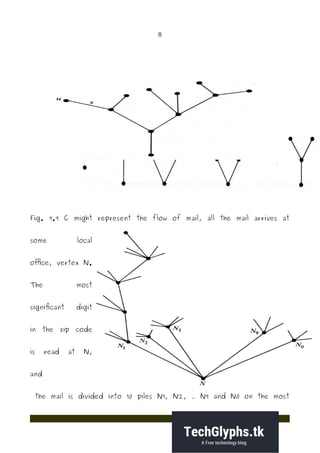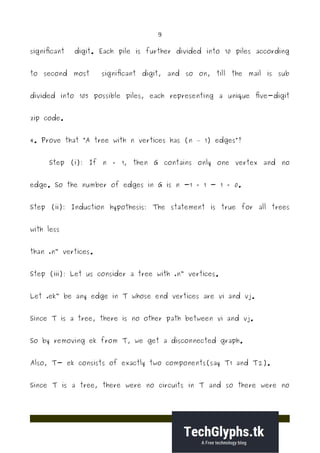Anúncio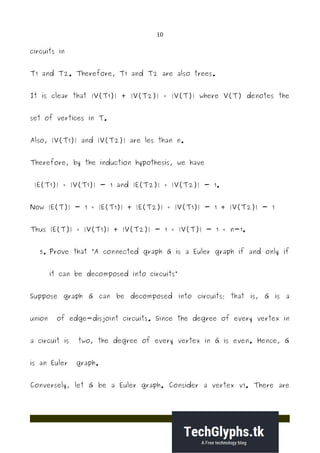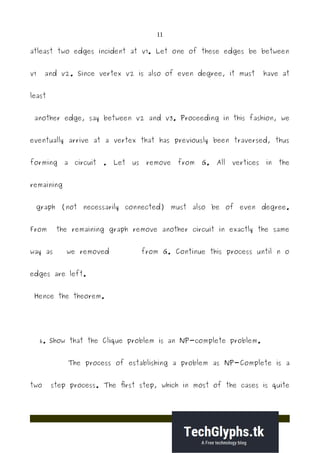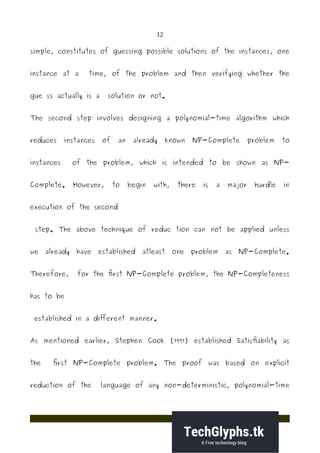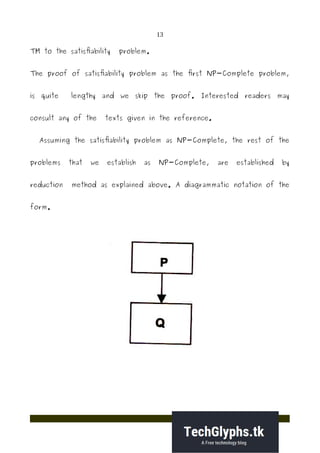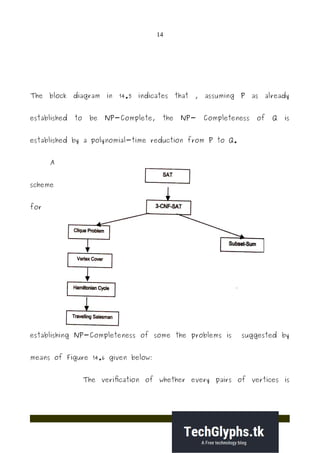Anúncio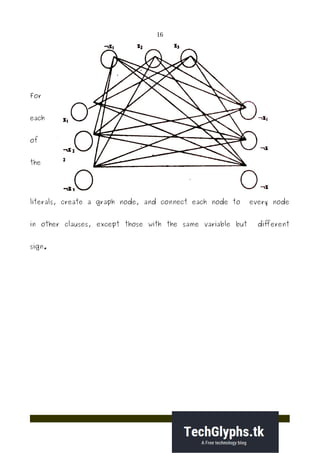Próximos SlideShares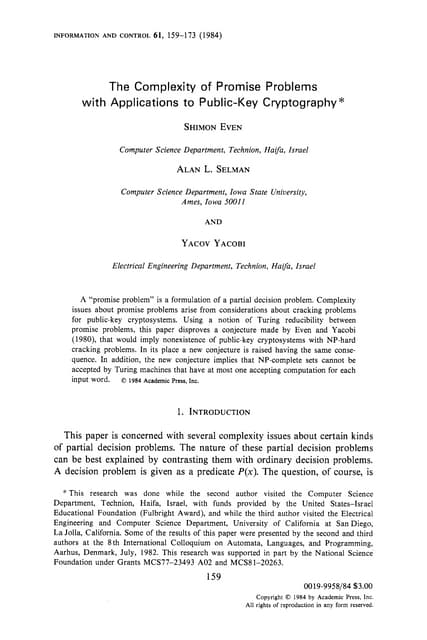The complexity of promise problems with applications to public-key cryptography
Carregando em ... 3
1 de 16
Anúncio

### Bt0080 fundamentals of algorithms2

1. 1 Fundamentals of Algorithms BT0080 Part-2 By Milan K Antony
2. 2 1.Write the steps involved in the general method for Branch and Bound? Explain? Branch and Bound strategy is a general algorithm for finding optimal solutions of various optimization problems, especially in discrete and combinatorial optimization. It consists of a systematic enumeration of all candidate solutions, where large subsets of fruitless candidates are discarded en masse, by using upper and lower estimated bounds of the quantity being optimized. In the following, we have n kinds of items, 1 through n. Each kind of item i has a value vi and a weight wi. We usually assume that all values and weights are nonnegative. To simplify the representation, we can also assume that the items are listed in increasing order of weight. The maximum weight that we can carry in the bag is W. The most common formulation of the problem is the 0-1 knapsack problem, which restricts the number xi of copies of each kind of item to zero or one. Mathematically the 0-1-knapsack problem can be formulated as:  maximize  subject to The bounded knapsack problem restricts the number xi of copies of each kind of item to a maximum integer value ci. Mathematically the bounded knapsack problem can be formulated as:  maximize  subject to The unbounded knapsack problem (UKP) places no upper bound on the number of copies of each kind of item.
3. 3 Of particular interest is the special case of the problem with these properties:  it is a decision problem,  it is a 0-1 problem,  for each kind of item, the weight equals the value: wi = vi. 1. Algorithm U Bound(cp, cw, k, m) 2. // cp is the current profit total, cw is the current 3. // weight total; k is the index of the last removed 4. // item; and m is the knapsack size 5. // w[i] and p[i] are respectively the weight and profit 6. // of the ith object 7. { 8. b:=cp; c:=cw; 9. for I:=k+1 to n do 10. { 11. if (c+w[i] m) then 12. { 13. c:c+w[i]; b:=b-p[i]; 14. } 15. } 16. return b; 17. } 18. 19. 20. One early application of knapsack algorithms was in the construction and scoring of tests in which the test-takers have a choice as to which questions they answer. On tests with a homogeneous distribution of point values for each question, it is a fairly simple process to provide the test-takers with such a choice. For example, if
4. 4 an exam contains 12 questions each worth 10 points, the test-taker need only answer 10 questions to achieve a maximum possible score of 100 points. However, on tests with a heterogeneous distribution of point values—that is, when different questions or sections are worth different amounts of points— it is more difficult to provide choices. Feuerman and Weiss proposed a system in which students are given a heterogeneous test with a total of 125 possible points. The students are asked to answer all of the questions to the best of their abilities. Of the possible subsets of problems whose total point values add up to 100, a knapsack algorithm would determine which subset gives each student the highest possible score 2. Explain the NP hard and the NP complete problems? The best algorithms for their solutions have computing times that cluster into two groups. The first group consists of problems whose solution are bounded by polynomials of small degree. The second group is made up to problems whose best-known algorithms are non-polynomial. Examples we have seen include the traveling salesperson and the knapsack problems for which the best algorithms given in this text have complexities O (n22n) and O (2n/2) respectively.
5. 5 In the quest to develop efficient algorithms, no one has been able to develop a polynomial time algorithm for any problem in the second group. This is very important because algorithms whose computing times are greater then, polynomial very quickly require such vast amounts of time to execute that even moderate-size problems cannot be solved. The theory of NP-completeness, which we present here, does not provide a method of obtaining polynomial time algorithms for problems in the second group. Nor does it say that algorithms of this complexity do not exist. Instead, what we do is show that many of the problems for which there are 4 no known polynomial time algorithms are computationally related. In fact, we establish two classes of problems. These are given names, NP-hard and NP-Complete. A problem that is NP-Complete has the property that it can be
6. 6 solved in polynomial time if and only if all other NP-Complete problems can also be solved in polynomial time. If an NP-hard problem can be solved in polynomial time, then all NP-Complete problems can be solved in polynomial time. All NP complete problems are NP-hard, but some NP-hard problems are not known to be NP-complete. Although one can define many distinct problem classes having the properties stated above for the NP- hard and NP-complete classes, the classes we study are related to non-deterministic computations. The relationship of these classes to non deterministic computations together with the apparent power of non- determinism lead to the intuitive conclusion that no NP- complete or NP- hard problem is polynomially solvable. 3. Briefly explain the concept of trees?
7. 7 A tree is a connected graph without any circuits. The graph in Fig. , for instance, is a tree. Trees with one, two, three and four vertices are shown in Fig. . A graph must have at least one vertex, and therefore so must a tree. Some authors allow the null tree, a tree without any vertices. It follows immediately from the definition that a tree has to be a simple graph, that is, having neither a self-loop nor parallel edges (because they both form circuits). Trees appear in numerous instances. The genealogy of a family is often represented by means of tree (in fact the term tree comes from family tree) A river with its tributaries and subtributaries can be represented by a tree. The sorting of mail according to zip code and the punched cards is done according to a tree (called decision tree or sorting tree).
8. 8 Fig. 9.9 C might represent the flow of mail, all the mail arrives at some local office, vertex N. The most significant digit in the zip code is read at N, and the mail is divided into 10 piles N1, N2, ... N9 and N0 on the most
9. 9 significant digit. Each pile is further divided into 10 piles according to second most significant digit, and so on, till the mail is sub divided into 105 possible piles, each representing a unique five-digit zip code. 4. Prove that “A tree with n vertices has (n – 1) edges”? Step (i): If n = 1, then G contains only one vertex and no edge. So the number of edges in G is n -1 = 1 - 1 = 0. Step (ii): Induction hypothesis: The statement is true for all trees with less than „n vertices.‟ Step (iii): Let us consider a tree with „n vertices.‟ Let „ek be any edge in T whose end vertices are vi and vj.‟ Since T is a tree, there is no other path between vi and vj. So by removing ek from T, we get a disconnected graph. Also, T- ek consists of exactly two components(say T1 and T2). Since T is a tree, there were no circuits in T and so there were no
10. 10 circuits in T1 and T2. Therefore, T1 and T2 are also trees. It is clear that |V(T1)| + |V(T2)| = |V(T)| where V(T) denotes the set of vertices in T. Also, |V(T1)| and |V(T2)| are les than n. Therefore, by the induction hypothesis, we have |E(T1)| = |V(T1)| - 1 and |E(T2)| = |V(T2)| - 1. Now |E(T)| - 1 = |E(T1)| + |E(T2)| = |V(T1)| - 1 + |V(T2)| - 1 Thus |E(T)| = |V(T1)| + |V(T2)| - 1 = |V(T)| - 1 = n-1. 5. Prove that “A connected graph G is a Euler graph if and only if it can be decomposed into circuits” Suppose graph G can be decomposed into circuits; that is, G is a union of edge-disjoint circuits. Since the degree of every vertex in a circuit is two, the degree of every vertex in G is even. Hence, G is an Euler graph. Conversely, let G be a Euler graph. Consider a vertex v1. There are
11. 11 atleast two edges incident at v1. Let one of these edges be between v1 and v2. Since vertex v2 is also of even degree, it must have at least another edge, say between v2 and v3. Proceeding in this fashion, we eventually arrive at a vertex that has previously been traversed, thus forming a circuit . Let us remove from G. All vertices in the remaining graph (not necessarily connected) must also be of even degree. From the remaining graph remove another circuit in exactly the same way as we removed from G. Continue this process until n o edges are left. Hence the theorem. 6. Show that the Clique problem is an NP-complete problem. The process of establishing a problem as NP-Complete is a two step process. The first step, which in most of the cases is quite
12. 12 simple, constitutes of guessing possible solutions of the instances, one instance at a time, of the problem and then verifying whether the gue ss actually is a solution or not. The second step involves designing a polynomial-time algorithm which reduces instances of an already known NP-Complete problem to instances of the problem, which is intended to be shown as NP- Complete. However, to begin with, there is a major hurdle in execution of the second step. The above technique of reduc tion can not be applied unless we already have established atleast one problem as NP-Complete. Therefore, for the first NP-Complete problem, the NP-Completeness has to be established in a different manner. As mentioned earlier, Stephen Cook (1971) established Satisfiability as the first NP-Complete problem. The proof was based on explicit reduction of the language of any non-deterministic, polynomial-time
13. 13 TM to the satisfiability problem. The proof of satisfiability problem as the first NP-Complete problem, is quite lengthy and we skip the proof. Interested readers may consult any of the texts given in the reference. Assuming the satisfiability problem as NP-Complete, the rest of the problems that we establish as NP-Complete, are established by reduction method as explained above. A diagrammatic notation of the form.
14. 14 The block diagram in 14.5 indicates that , assuming P as already established to be NP-Complete, the NP- Completeness of Q is established by a polynomial-time reduction from P to Q. A scheme for establishing NP-Completeness of some the problems is suggested by means of Figure 14.6 given below: The verification of whether every pairs of vertices is
15. 15 connected by an edge in E, is done for different pairs of vertices by a Non-deterministic TM, i.e., in parallel. Hence, it takes only polynomial time because for each of n vertices we need to verify atmost n (n + 1) /2 edges, the maximum number of edges in a graph with n vertices. Next, we show that 3-CNF-SAT problem can be transformed to clique problem in polynomial time. Take an instance of 3-CNF-SAT. An instance of 3CNF-SAT consists of a set of n clauses, each consisting of exactly 3 literal, each being either a variable or negated variable. It is satisfiable if we can choose literals in such a way that: • Atleast one literal from each clause is chosen • If literal of form x is chosen, no literal of form x is considered.
16. 16 For each of the literals, create a graph node, and connect each node to every node in other clauses, except those with the same variable but different sign.
Anúncio